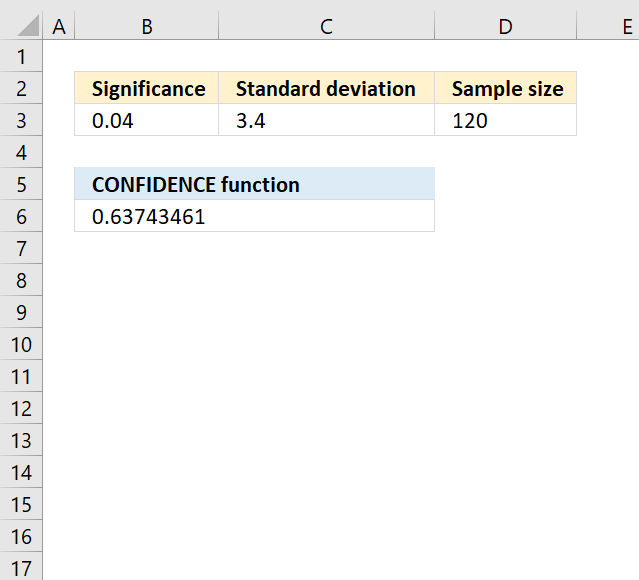Author: Oscar Cronquist Article last updated on May 11, 2022The CONFIDENCE function calculates the confidence interval for a population mean, using a normal distribution.

Formula in cell B6:

=CONFIDENCE(B3, C3, D3)

### Excel Function Syntax

CONFIDENCE(alpha, standard_dev, size)

### Arguments

 alpha Required. The significance level used to calculate the confidence level. 0.05 represents a 95% confidence level. standard_dev Required. The standard deviation for the data points. size Required. The number of data points.